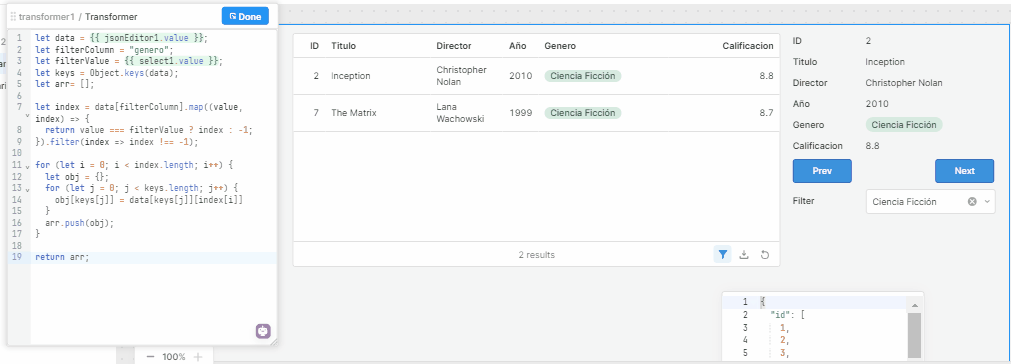# Access table items after applying filters

Hello,

Is it possible to loop through visible items in tables after applying a filter? I can't find any table method or property that would allow me to do something like that.

Hello, You can instead have a transformer with the data source and apply the filter to that transformer

Here is my approach``````let data = {{ jsonEditor1.value }};
let filterColumn = "genero";
let filterValue = {{ select1.value }};
let keys = Object.keys(data);
let arr= [];

let index = data[filterColumn].map((value, index) => {
return value === filterValue ? index : -1;
}).filter(index => index !== -1);

for (let i = 0; i < index.length; i++) {
let obj = {};
for (let j = 0; j < keys.length; j++) {
obj[keys[j]] = data[keys[j]][index[i]]
}
arr.push(obj);
}

return arr;
``````

Thanks for the idea, but I need to approach it in different way. I need to have access to all rows which are visible in the table after user applies filters. I don't want to create custom filtering logic - I have Retool table for it.

Check out this post

2 Likes

Thank you @ScottR, that's exactly what I need!